# Signal Processing: Reconstructing Signal using Sinc Interpolation

in STEMGeeks2 months ago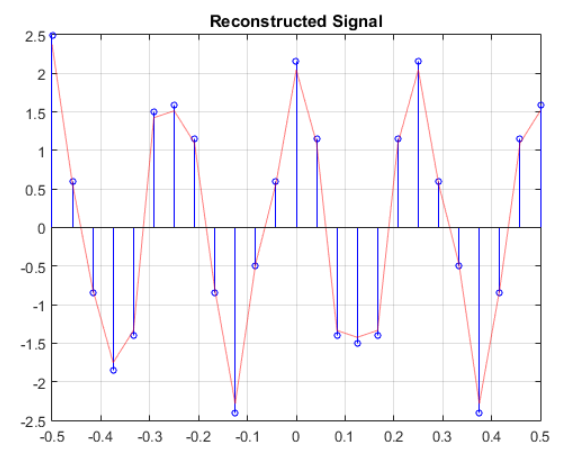#### 1. Introduction

A reconstruction filter is used after the output of the D/A system. It is a low pass filter, which removes all the high frequency component of the signal. We consider a "stairstep" reconstructions and remove the "jagged" edges to reconstruct the signal. The filter has a cut-off frequency of fs/2. The signal of interest is band limited with anti-aliasing input filter. We sampled the signal in accordance to the Nyquist rate. We used interpolation to reconstruct the signal. In the next section, the reconstruction and MATLAB implementation is discussed.

#### 2. Ideal reconstruction in MATLAB

The “ sinc ” function sin(πt/T)/(πt/T ) for the ideal reconstruction of a sample at t = 0, with a sampling period of T = 0.1. The reconstruction function h ( t ), the zero crossings are at nT , where n is an integer. We can say the function is zero when π/T is 0, π, 2π, ... which occurs at t = nT for any integer n. The reconstruction is simply a sampled-weighted Sinc function. The Sinc function provides an optimal interpolation.

The number of interpolation functions must be overlaid, one at each sample instant. We also get the weighted value of the sample at that instant. For this blog, we consider a discrete signal represented by

xn = 2cos(2πf1n)+0.5cos(2πf2n).

The value of f1 = 1/6 and f2 = 2/5. We sampled the signal and use Sinc interpolation to reconstruct the signal. Also, we present the interpolation plot at each sample.

We start by setting the sample frequency and time based from f1 and f2 values. At the same time, we establish the time and samples. We initialize our code with

``````close all; clear all; clc;
f1 = 1/6; f2 = 2/5;
N = 24;
Fs = 10*(f2/f1);
Ts = 1/Fs;
t = -0.5:Ts:0.5;
n = 0:1:N;
xn = 2*cos(2*pi*f1*n)+0.5*cos(2*pi*f2*n);
``````

Next we set the sinc function by substituting the sampling time to it. Afterwards, we present the sinc plot which is the basis for our interpolation.

``````for i = 1:length(t)
h(i) = sinc(pi*t(i)/Ts);
end
figure;
plot(t,h); grid on;
``````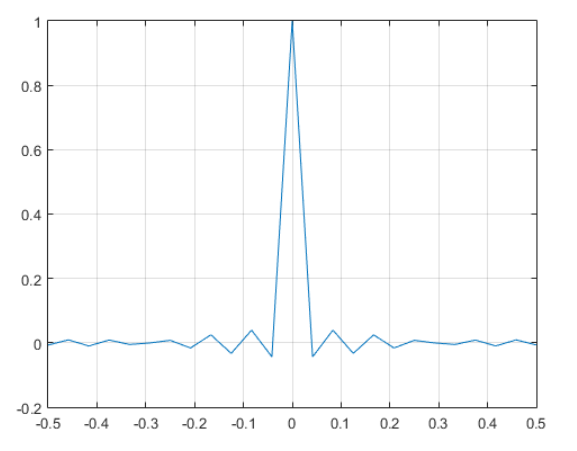Sinc Function

We multiply the sinc function to our signal to interpolate. Then, we sum up all interpolation function for each samples to generate the reconstructed signal.

``````xt = 0;
Xt = [];
sum_Xt = 0;
for j = 1:length(n)
xt = xn*h(j);
sum_Xt = sum_Xt+xt;
Xt = [Xt; {xt}];
figure;
plot(t, Xt{j}); hold on;grid on;
xlabel('Time'); ylabel('Amplitude');
title(sprintf('Interpolation Function (n = %d)',j-1));
end
``````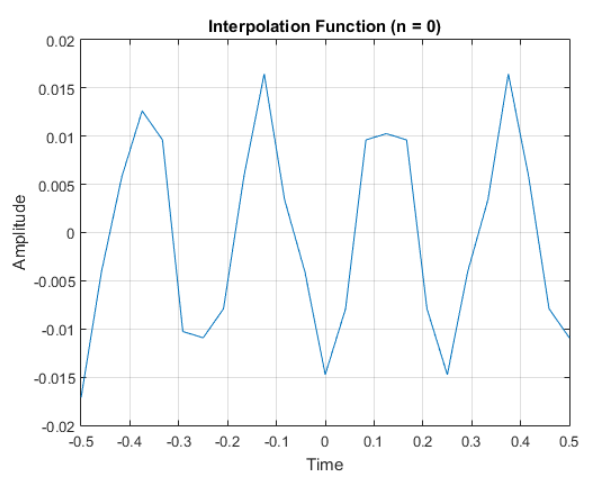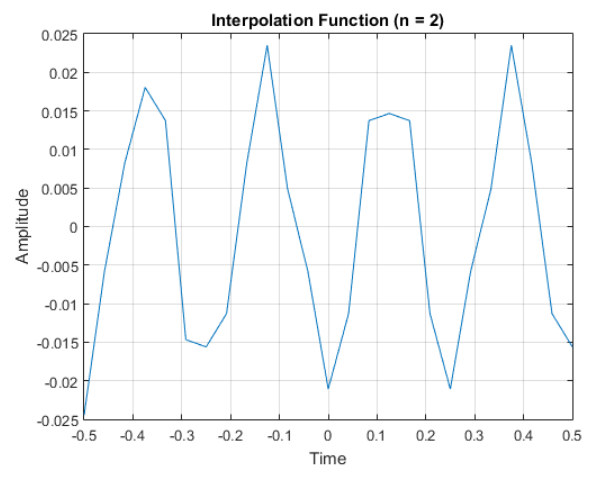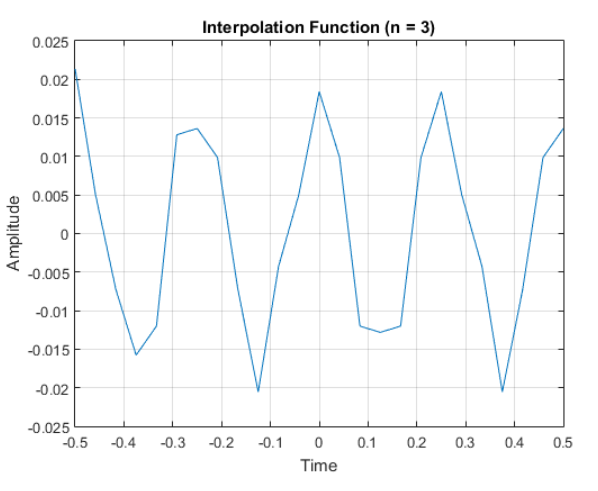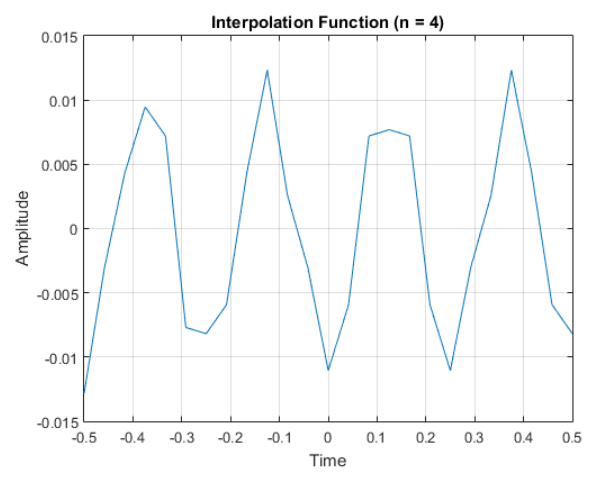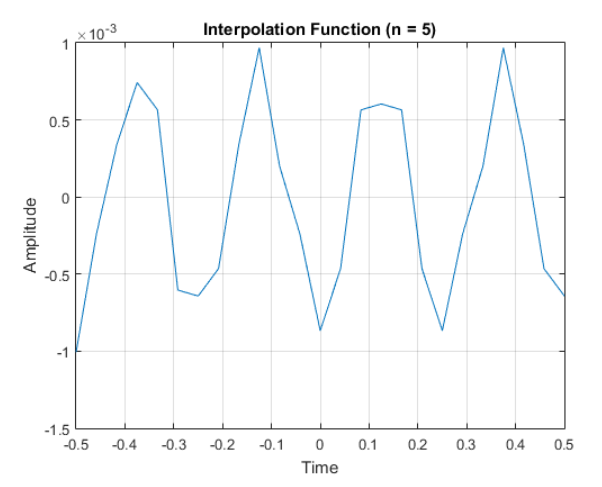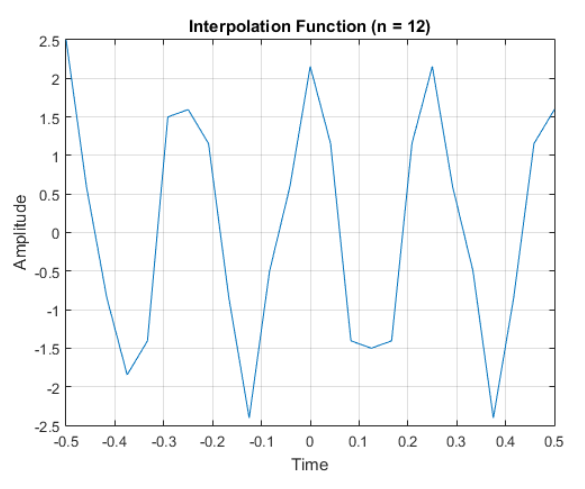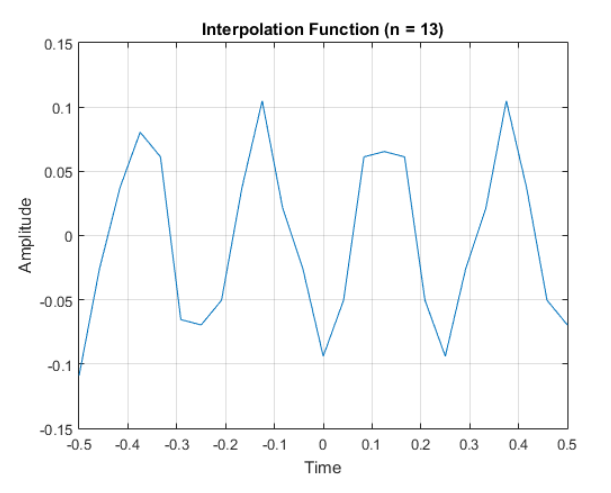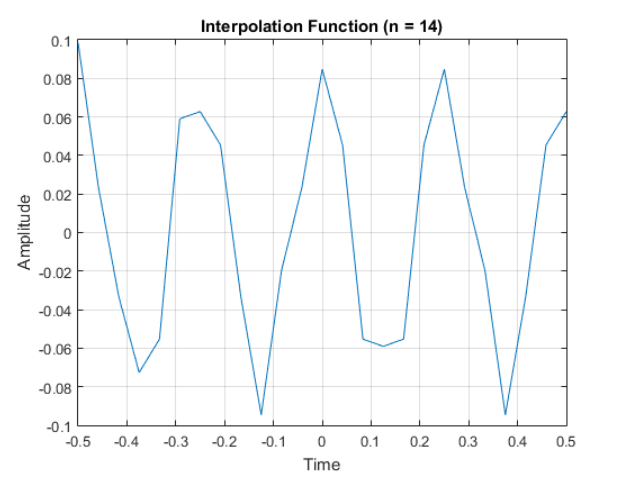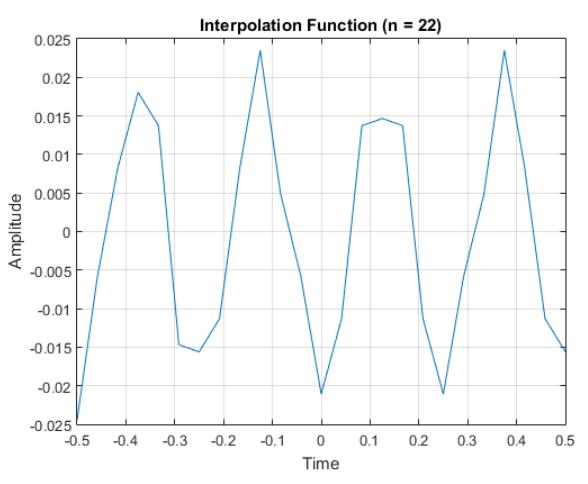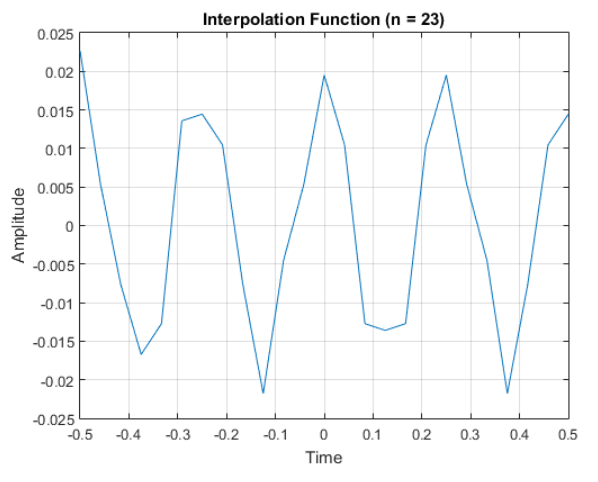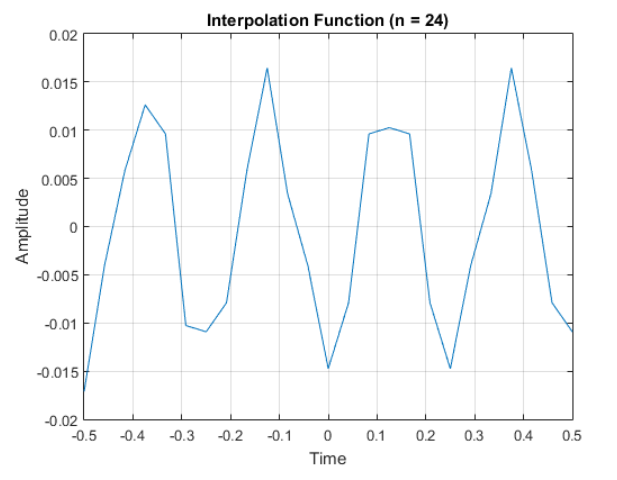The plot shown above are samples from the generated interpolation function and values. When we combine all of the values we can see the sum of this values.

``````figure;
for k = 1:length(n)
pol = plot(t, Xt{k}); hold on;grid on;
pol.Color = [pol.Color 0.5];
xlabel('Time'); ylabel('Amplitude');
plot(t, sum_Xt,'--','LineWidth',1); grid on;
end
``````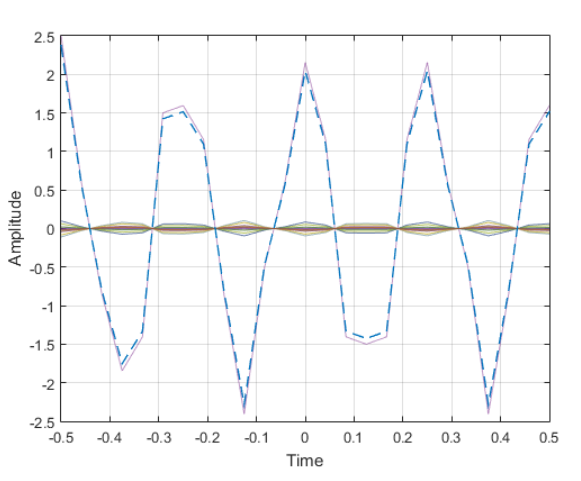Interpolation Function

The dashed line represent the summation of the interpolation function. At the same time, it represent the reconstructed signal from the discrete-time signal xn = 2cos(2πf1n)+0.5cos(2πf2n). The reconstructed signal is shown in details in the next plots.

``````figure;
ax1 = subplot(2,1,1);
stem(t,xn, 'b','MarkerSize', 4); hold on; grid on;
ax2 = subplot(2,1,2);
plot(t,sum_Xt, 'r');hold on; grid on;
xlabel(ax1, 'samples');ylabel(ax1, 'levels');
title(ax1, 'Discrete-time Signal');
xlabel(ax2, 'time');ylabel(ax2, 'amplitude');
title(ax2, 'Continuous-time Signal (Reconstructed)')
``````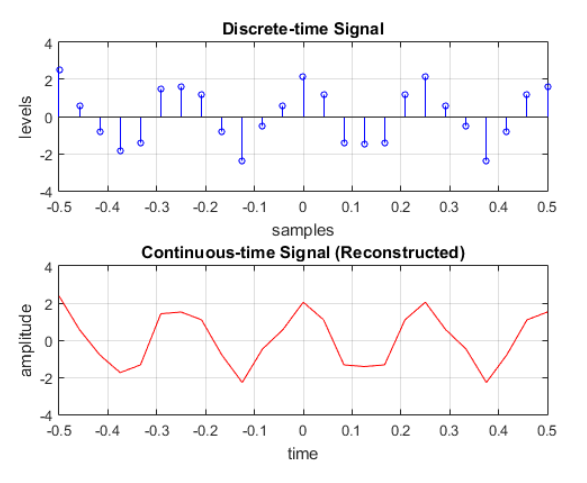The reconstructed signal has a minimal error as compared to the original signal. It is due to the error innate in interpolating the signal and due to quantization of the original signal to a discrete one. The reconstructed signal plotted against the discrete-time signal has values that are lower than the original discrete signal.#### 3. Conclusion

A reconstruction filter enables us to revert the discrete values into its continuous-time values. In this blog, we used a Sinc function to interpolate the discrete values to reconstruct the original signal. The reconstruction of a signal may not be accurate however it still represents the original continuous-time signal.

### 4. References

1. Gérard Blanchet, Maurice Charbit, Digital Signal and Image Processing Using MATLAB, online access

2. Neal S. Widmer, Gregory L. Moss, Ronald J. Tocci, Digital Systems: Principles and Applications, 12th Edition, online access

3. John W. Leis, Digital Signal Processing Using MATLAB for Students and Researchers. online access

Posted with STEMGeeks

Sort: